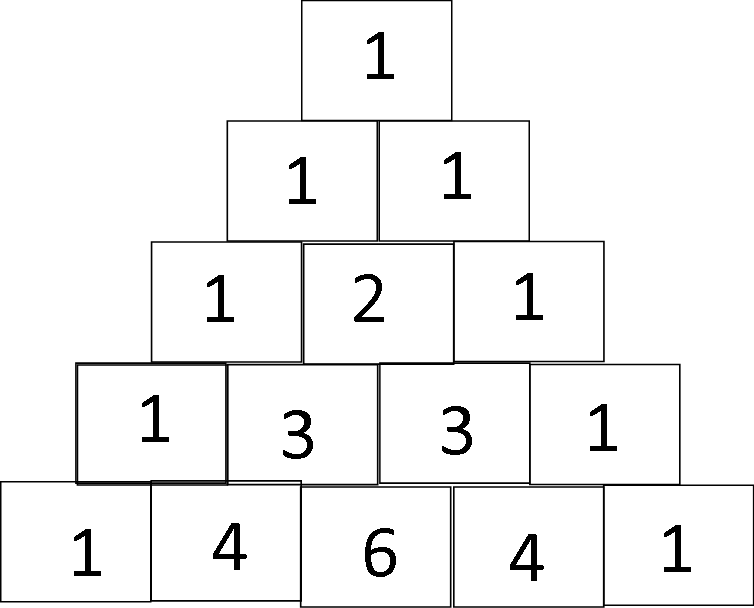# Pascal's Triangle: Definition and Use with Polynomials

An error occurred trying to load this video.

Try refreshing the page, or contact customer support.

Coming up next: The Binomial Theorem: Defining Expressions

### You're on a roll. Keep up the good work!

Replay
Your next lesson will play in 10 seconds
• 0:03 Pascal's Triangle
• 1:51 Polynomial Uses
• 4:06 Examples
• 6:32 Lesson Summary
Save Save

Want to watch this again later?

Timeline
Autoplay
Autoplay
Speed Speed Audio mode

#### Recommended Lessons and Courses for You

Lesson Transcript
Instructor: Maria Airth

Maria has a Doctorate of Education and over 20 years of experience teaching psychology and math related courses at the university level.

Pascal's Triangle is defined and discussed briefly. Following the introduction to the triangle, its use in expanding polynomial powers is elaborated with examples.

## Pascal's Triangle

Have you ever been to Egypt? I haven't, but it is definitely one of the places in this world that I would like to visit. Thinking of Egypt always brings to mind pyramids! Pyramids evoke a sense of mystery and amazement in me.

In this lesson, we will be learning about the mysterious and amazing Pascal's Triangle. Pascal's Triangle is an amazing number pattern that creates a pyramid, or triangle, shape out of the binomial coefficients. It is named after the French mathematician.

The triangle starts with a 1 at the top, the pinnacle of the pyramid. The next line in the triangle has two 1s: like this:The third line of the triangle is 1, 2, 1. This is where it starts to get really interesting. Let's put in two more lines of the triangle to really see what is going on.

Did you notice that the outside edges are always 1? Did you notice that inside the triangle, each new number is found by adding the two above it?

If you didn't notice the adding aspect, look again. On the second line we have 1 and 1. Just under those two ones is a 2. 1 + 1 = 2. On the next line down we have a 1 and a 2 next to each other. Under them is a 3. Under the two 3s is a 6. Do you see it now? This pattern continues for the whole triangle. Using this adding process, you could create Pascal's Triangle for yourself out to however many lines you'd like.

The last thing to notice, and it will be even more obvious as you build the triangle, is that the triangle is perfectly symmetrical. So, as you are building the lines, remember, once you reach the middle, the rest of the numbers for any line will match the reverse of the first half of the line.

## Polynomial Uses For Pascal's Triangle

Okay, so, Pascal's Triangle is a pretty cool pattern of numbers, but how does that help with polynomials?

Before we answer that, let's look at a polynomial expansion: (x + 1)^3. In order to work out this problem we have to multiply: (x + 1)(x + 1)(x + 1). Eventually, we would end up with: x^3 + 3x^2 + 3x + 1.

If you aren't sure how I got that answer, please review some of the other algebra lessons.

• Starting with the original power, the powers decrease by one in each term
• The coefficients for each term are 1, 3, 3, 1

Wait, 1, 3, 3, 1! That is one of the lines of the triangle!

Are you ready for this? Each line in the triangle actually represents the coefficients of a polynomial expansion!

Looking back at our triangle, notice how the polynomial expansion coefficients match up perfectly with the triangle numbers:

• (x + 1)^0 = 1
• (x + 1)^1 = 1x + 1
• (x + 1)^2 = 1x^2 + 2x + 1
• (x + 1)^3 = 1x^3 + 3x^2 + 3x + 1
• (x + 1)^4 = 1x^4 + 4x^3 + 6x^2 + 4x + 1

This illustration makes the pattern pretty easy to see. If you wanted to expand (x + 1)^5, you would just need to add another line to the triangle: 1, 5, 10, 10, 5, 1. Thus the expansion is x^5 + 5x^4 + 10x^3 + 10x^2 + 5x + 1.

All you have to remember is to decrease the exponents for each term, and the rest is done for you in the triangle!

To unlock this lesson you must be a Study.com Member.

### Register to view this lesson

Are you a student or a teacher?

#### See for yourself why 30 million people use Study.com

##### Become a Study.com member and start learning now.
Back
What teachers are saying about Study.com

### Earning College Credit

Did you know… We have over 200 college courses that prepare you to earn credit by exam that is accepted by over 1,500 colleges and universities. You can test out of the first two years of college and save thousands off your degree. Anyone can earn credit-by-exam regardless of age or education level.